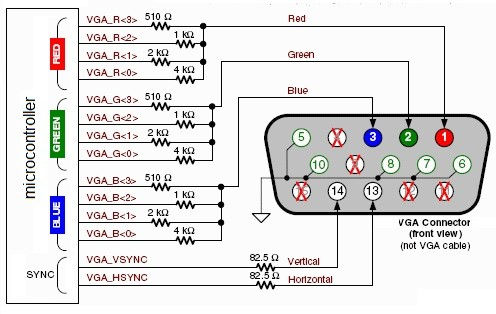# Strange VGA Effects on an FPGA

When I assembled my VGA implementation on my Mojo FPGA, I did so with only a single wire for each of the color signals (Red, Green, and Blue). This limited my color choices to 8 colors. I read about using Pulse width modulation to send other values to a wire, so I gave it a try. The results were interesting, but not what I was looking for.

Here is a video of the results:

Here is my VHDL code:

``````----------------------------------------------------------------------------------
-- Company:
-- Engineer:
--
-- Create Date:    04:37:23 05/27/2017
-- Design Name:
-- Module Name:    img_gen - Behavioral
-- Project Name:
-- Target Devices:
-- Tool versions:
-- Description:
--
-- Dependencies:
--
-- Revision:
-- Revision 0.01 - File Created
--
----------------------------------------------------------------------------------
library IEEE;
use IEEE.STD_LOGIC_1164.ALL;
use IEEE.STD_LOGIC_ARITH.ALL;
use IEEE.STD_LOGIC_UNSIGNED.ALL;

entity img_gen is
Port ( clk : in STD_LOGIC;
x_control : in STD_LOGIC_VECTOR(9 downto 0);
paddle : in STD_LOGIC_VECTOR(9 downto 0);
y_control : in STD_LOGIC_VECTOR(9 downto 0);
video_on : in STD_LOGIC;
rgb : out STD_LOGIC_VECTOR(2 downto 0));
end img_gen;

architecture Behavioral of img_gen is

signal  PWM_R_Accumulator : std_logic_vector(8 downto 0);
signal  PWM_G_Accumulator : std_logic_vector(8 downto 0);
signal  PWM_B_Accumulator : std_logic_vector(8 downto 0);

--wall
constant wall_l:integer :=10;--the distance between wall and left side of screen
constant wall_t:integer :=10;--the distance between wall and top side of screen
constant wall_k:integer :=10;--wall thickness
signal wall_on:std_logic;
signal rgb_wall:std_logic_vector(23 downto 0);

--bar
signal bar_l, bar_l_next: integer:=100;
constant bar_t:integer :=420;--the distance between bar and top side of screen
constant bar_k:integer :=10;--bar thickness
constant bar_w:integer:=120;--bar width
constant bar_v:integer:=10;--velocity of the bar
signal bar_on:std_logic;
signal rgb_bar:std_logic_vector(23 downto 0);

--ball
signal ball_l,ball_l_next:integer :=100;--the distance between ball and left side of screen
signal ball_t,ball_t_next:integer :=100; --the distance between ball and top side of screen
constant ball_w:integer :=20;--ball Height
constant ball_u:integer :=20;--ball width
constant x_v,y_v:integer:=3;-- horizontal and vertical speeds of the ball
signal ball_on:std_logic;
signal rgb_ball:std_logic_vector(23 downto 0);

--refreshing(1/60)
signal refresh_reg,refresh_next:integer;
constant refresh_constant:integer:=830000;
signal refresh_tick:std_logic;

--ball animation
signal xv_reg,xv_next:integer:=3;--variable of the horizontal speed
signal yv_reg,yv_next:integer:=3;--variable of the vertical speed

--x,y pixel cursor
signal x,y:integer range 0 to 650;

--mux
signal vdbt:std_logic_vector(3 downto 0);

--buffer
signal rgb_reg:std_logic_vector(2 downto 0);
signal rgb_next:std_logic_vector(23 downto 0);

begin

--x,y pixel cursor
x <=conv_integer(x_control);
y <=conv_integer(y_control );

--refreshing
process(clk)
begin
if clk'event and clk='1' then
refresh_reg<=refresh_next;
end if;
end process;
refresh_next<= 0 when refresh_reg= refresh_constant else
refresh_reg+1;
refresh_tick<= '1' when refresh_reg = 0 else
'0';
--register part
process(clk)
begin
if clk'event and clk='1' then
ball_l<=ball_l_next;
ball_t<=ball_t_next;
xv_reg<=xv_next;
yv_reg<=yv_next;
bar_l<=bar_l_next;
end if;
end process;

--bar animation
begin

if refresh_tick= '1' then
end if;
end process;

--ball animation
process(refresh_tick,ball_l,ball_t,xv_reg,yv_reg)
begin
ball_l_next <=ball_l;
ball_t_next <=ball_t;
xv_next<=xv_reg;
yv_next<=yv_reg;
if refresh_tick = '1' then
if ball_t> 400 and ball_l > (bar_l -ball_u) and ball_l < (bar_l +120) then --the ball hits the bar
yv_next<= -y_v ;
elsif ball_t< 35 then--The ball hits the wall
yv_next<= y_v;
end if;
if ball_l < 10 then --The ball hits the left side of the screen
xv_next<= x_v;
elsif ball_l> 600 then
xv_next<= -x_v ; --The ball hits the right side of the screen
end if;
ball_l_next <=ball_l +xv_reg;
ball_t_next <=ball_t+yv_reg;
end if;
end process;

--wall object
wall_on <= '1' when x > wall_l and x < (640-wall_l) and y> wall_t and y < (wall_t+ wall_k) else
'0';
rgb_wall<=x"010101";--Black
--bar object
bar_on <= '1' when x > bar_l and x < (bar_l+bar_w) and y> bar_t and y < (bar_t+ bar_k) else
'0';
rgb_bar<=x"0000F1";--blue

--ball object
ball_on <= '1' when x > ball_l and x < (ball_l+ball_u) and y> ball_t and y < (ball_t+ ball_w) else
'0';
rgb_ball<=x"00F100"; --Green

--buffer
process(clk)
begin
if clk'event and clk='1' then
PWM_R_Accumulator  <=  ("0" & PWM_R_Accumulator(7 downto 0)) + ("0" & rgb_next(23 downto 16));
PWM_G_Accumulator  <=  ("0" & PWM_G_Accumulator(7 downto 0)) + ("0" & rgb_next(15 downto 8));
PWM_B_Accumulator  <=  ("0" & PWM_B_Accumulator(7 downto 0)) + ("0" & rgb_next(7 downto 0));
end if;
if clk'event and clk='1' then
--rgb_reg<=rgb_next;

end if;
end process;

--mux
vdbt<=video_on & wall_on & bar_on &ball_on;
with vdbt select
rgb_next <= x"FFAAAA" when "1000",--Background of the screen is red
rgb_wall when "1100",
rgb_wall when "1101",
rgb_bar when "1010",
rgb_bar when "1011",
rgb_ball when "1001",
x"000000" when others;
--output

rgb<=PWM_R_Accumulator(8) & PWM_G_Accumulator(8) & PWM_B_Accumulator(8);

end Behavioral;
``````

I added three new signals for the PWMs. One PWM per color. Those are defined starting on line 37. Once those were defined, I then set the colors on the objects on the screen (the wall, the ball, the paddle, and the background). That code starts on line 149 (colors are defined in hexadecimal).

I then defaulted the color to red for things that didn't have a setting (line 178).

On line 187, I set the output of the VGA wires to the most significant bit of the PWM accumulators.

The idea was that the PWM would send a different color to the monitor. I really don't know how (or even if) I can fix it, but the results are interesting.

After watching the video below, I learned that this is a hardware issue. I need to build out the different resistance values to handle each of the bits with a gpio pin on the FPGA.

Here is a 12-bit schematic that I'm going to try next.(image source: https://electronics.stackexchange.com/questions/228825/programming-pattern-to-generate-vga-signal-with-micro-controller)